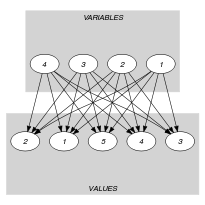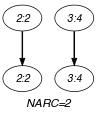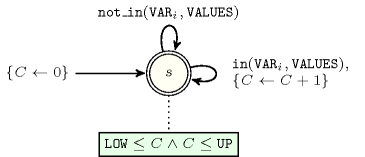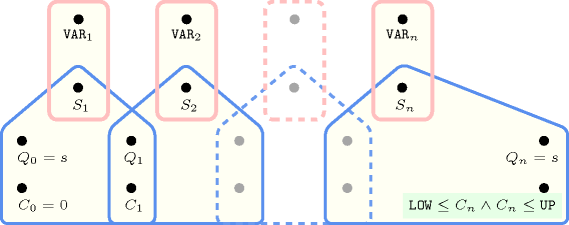## 5.26. among_low_up

Origin
Constraint

$\mathrm{𝚊𝚖𝚘𝚗𝚐}_\mathrm{𝚕𝚘𝚠}_\mathrm{𝚞𝚙}\left(\mathrm{𝙻𝙾𝚆},\mathrm{𝚄𝙿},\mathrm{𝚅𝙰𝚁𝙸𝙰𝙱𝙻𝙴𝚂},\mathrm{𝚅𝙰𝙻𝚄𝙴𝚂}\right)$

Arguments
 $\mathrm{𝙻𝙾𝚆}$ $\mathrm{𝚒𝚗𝚝}$ $\mathrm{𝚄𝙿}$ $\mathrm{𝚒𝚗𝚝}$ $\mathrm{𝚅𝙰𝚁𝙸𝙰𝙱𝙻𝙴𝚂}$ $\mathrm{𝚌𝚘𝚕𝚕𝚎𝚌𝚝𝚒𝚘𝚗}\left(\mathrm{𝚟𝚊𝚛}-\mathrm{𝚍𝚟𝚊𝚛}\right)$ $\mathrm{𝚅𝙰𝙻𝚄𝙴𝚂}$ $\mathrm{𝚌𝚘𝚕𝚕𝚎𝚌𝚝𝚒𝚘𝚗}\left(\mathrm{𝚟𝚊𝚕}-\mathrm{𝚒𝚗𝚝}\right)$
Restrictions
 $\mathrm{𝙻𝙾𝚆}\ge 0$ $\mathrm{𝙻𝙾𝚆}\le |\mathrm{𝚅𝙰𝚁𝙸𝙰𝙱𝙻𝙴𝚂}|$ $\mathrm{𝚄𝙿}\ge 0$ $\mathrm{𝚄𝙿}\le |\mathrm{𝚅𝙰𝚁𝙸𝙰𝙱𝙻𝙴𝚂}|$ $\mathrm{𝚄𝙿}\ge \mathrm{𝙻𝙾𝚆}$ $\mathrm{𝚛𝚎𝚚𝚞𝚒𝚛𝚎𝚍}$$\left(\mathrm{𝚅𝙰𝚁𝙸𝙰𝙱𝙻𝙴𝚂},\mathrm{𝚟𝚊𝚛}\right)$ $\mathrm{𝚛𝚎𝚚𝚞𝚒𝚛𝚎𝚍}$$\left(\mathrm{𝚅𝙰𝙻𝚄𝙴𝚂},\mathrm{𝚟𝚊𝚕}\right)$ $\mathrm{𝚍𝚒𝚜𝚝𝚒𝚗𝚌𝚝}$$\left(\mathrm{𝚅𝙰𝙻𝚄𝙴𝚂},\mathrm{𝚟𝚊𝚕}\right)$
Purpose

Between $\mathrm{𝙻𝙾𝚆}$ and $\mathrm{𝚄𝙿}$ variables of the $\mathrm{𝚅𝙰𝚁𝙸𝙰𝙱𝙻𝙴𝚂}$ collection are assigned a value of the $\mathrm{𝚅𝙰𝙻𝚄𝙴𝚂}$ collection.

Example
$\left(1,2,〈9,2,4,5〉,〈0,2,4,6,8〉\right)$

The $\mathrm{𝚊𝚖𝚘𝚗𝚐}_\mathrm{𝚕𝚘𝚠}_\mathrm{𝚞𝚙}$ constraint holds since between 1 and 2 values (i.e., in fact 2 values) of the collection of values $〈9,2,4,5〉$ belong to the set of values $\left\{0,2,4,6,8\right\}$.

Typical
 $\mathrm{𝙻𝙾𝚆}<|\mathrm{𝚅𝙰𝚁𝙸𝙰𝙱𝙻𝙴𝚂}|$ $\mathrm{𝚄𝙿}>0$ $\mathrm{𝙻𝙾𝚆}<\mathrm{𝚄𝙿}$ $|\mathrm{𝚅𝙰𝚁𝙸𝙰𝙱𝙻𝙴𝚂}|>1$ $|\mathrm{𝚅𝙰𝙻𝚄𝙴𝚂}|>1$ $|\mathrm{𝚅𝙰𝚁𝙸𝙰𝙱𝙻𝙴𝚂}|>|\mathrm{𝚅𝙰𝙻𝚄𝙴𝚂}|$ $\mathrm{𝙻𝙾𝚆}>0\vee \mathrm{𝚄𝙿}<|\mathrm{𝚅𝙰𝚁𝙸𝙰𝙱𝙻𝙴𝚂}|$
Symmetries
• Items of $\mathrm{𝚅𝙰𝚁𝙸𝙰𝙱𝙻𝙴𝚂}$ are permutable.

• Items of $\mathrm{𝚅𝙰𝙻𝚄𝙴𝚂}$ are permutable.

• $\mathrm{𝙻𝙾𝚆}$ can be decreased to any value $\ge 0$.

• $\mathrm{𝚄𝙿}$ can be increased to any value $\le |\mathrm{𝚅𝙰𝚁𝙸𝙰𝙱𝙻𝙴𝚂}|$.

• An occurrence of a value of $\mathrm{𝚅𝙰𝚁𝙸𝙰𝙱𝙻𝙴𝚂}.\mathrm{𝚟𝚊𝚛}$ that belongs to $\mathrm{𝚅𝙰𝙻𝚄𝙴𝚂}.\mathrm{𝚟𝚊𝚕}$ (resp. does not belong to $\mathrm{𝚅𝙰𝙻𝚄𝙴𝚂}.\mathrm{𝚟𝚊𝚕}$) can be replaced by any other value in $\mathrm{𝚅𝙰𝙻𝚄𝙴𝚂}.\mathrm{𝚟𝚊𝚕}$ (resp. not in $\mathrm{𝚅𝙰𝙻𝚄𝙴𝚂}.\mathrm{𝚟𝚊𝚕}$).

Arg. properties
• Contractible wrt. $\mathrm{𝚅𝙰𝚁𝙸𝙰𝙱𝙻𝙴𝚂}$ when $\mathrm{𝚄𝙿}=0$.

• Contractible wrt. $\mathrm{𝚅𝙰𝚁𝙸𝙰𝙱𝙻𝙴𝚂}$ when $\mathrm{𝚄𝙿}=|\mathrm{𝚅𝙰𝚁𝙸𝙰𝙱𝙻𝙴𝚂}|$.

• Aggregate: $\mathrm{𝙻𝙾𝚆}\left(+\right)$, $\mathrm{𝚄𝙿}\left(+\right)$, $\mathrm{𝚅𝙰𝚁𝙸𝙰𝙱𝙻𝙴𝚂}\left(\mathrm{𝚞𝚗𝚒𝚘𝚗}\right)$, $\mathrm{𝚅𝙰𝙻𝚄𝙴𝚂}\left(\mathrm{𝚜𝚞𝚗𝚒𝚘𝚗}\right)$.

Algorithm

The $\mathrm{𝚊𝚖𝚘𝚗𝚐}_\mathrm{𝚕𝚘𝚠}_\mathrm{𝚞𝚙}$ constraint is entailed if and only if the following two conditions hold:

1. The number of variables of the $\mathrm{𝚅𝙰𝚁𝙸𝙰𝙱𝙻𝙴𝚂}$ collection assigned a value of the $\mathrm{𝚅𝙰𝙻𝚄𝙴𝚂}$ collection is greater than or equal to $\mathrm{𝙻𝙾𝚆}$.

2. The number of variables of the $\mathrm{𝚅𝙰𝚁𝙸𝙰𝙱𝙻𝙴𝚂}$ collection that can potentially be assigned a value of the $\mathrm{𝚅𝙰𝙻𝚄𝙴𝚂}$ collection is less than or equal to $\mathrm{𝚄𝙿}$.

Used in

assignment dimension added: $\mathrm{𝚒𝚗𝚝𝚎𝚛𝚟𝚊𝚕}_\mathrm{𝚊𝚗𝚍}_\mathrm{𝚌𝚘𝚞𝚗𝚝}$ (assignment dimension corresponding to intervals added).

generalisation: $\mathrm{𝚊𝚖𝚘𝚗𝚐}$ ($\mathrm{𝚒𝚗𝚝𝚎𝚛𝚟𝚊𝚕}$ replaced by $\mathrm{𝚟𝚊𝚛𝚒𝚊𝚋𝚕𝚎}$), $\mathrm{𝚜𝚕𝚒𝚍𝚒𝚗𝚐}_\mathrm{𝚌𝚊𝚛𝚍}_\mathrm{𝚜𝚔𝚒𝚙}\mathtt{0}$ (full sequence replaced by maximal sequences of non-zeros).

Keywords
Cond. implications

$\mathrm{𝚊𝚖𝚘𝚗𝚐}_\mathrm{𝚕𝚘𝚠}_\mathrm{𝚞𝚙}\left(\mathrm{𝙻𝙾𝚆},\mathrm{𝚄𝙿},\mathrm{𝚅𝙰𝚁𝙸𝙰𝙱𝙻𝙴𝚂},\mathrm{𝚅𝙰𝙻𝚄𝙴𝚂}\right)$

with  $\mathrm{𝚍𝚒𝚜𝚝𝚒𝚗𝚌𝚝}$$\left(\mathrm{𝚅𝙰𝚁𝙸𝙰𝙱𝙻𝙴𝚂},\mathrm{𝚟𝚊𝚛}\right)$

implies $\mathrm{𝚊𝚖𝚘𝚗𝚐}_\mathrm{𝚕𝚘𝚠}_\mathrm{𝚞𝚙}\left(\mathrm{𝙻𝙾𝚆},\mathrm{𝚄𝙿},\mathrm{𝚅𝙰𝙻𝚄𝙴𝚂},\mathrm{𝚅𝙰𝚁𝙸𝙰𝙱𝙻𝙴𝚂}\right)$.

Arc input(s)

$\mathrm{𝚅𝙰𝚁𝙸𝙰𝙱𝙻𝙴𝚂}$ $\mathrm{𝚅𝙰𝙻𝚄𝙴𝚂}$

Arc generator
$\mathrm{𝑃𝑅𝑂𝐷𝑈𝐶𝑇}$$↦\mathrm{𝚌𝚘𝚕𝚕𝚎𝚌𝚝𝚒𝚘𝚗}\left(\mathrm{𝚟𝚊𝚛𝚒𝚊𝚋𝚕𝚎𝚜},\mathrm{𝚟𝚊𝚕𝚞𝚎𝚜}\right)$

Arc arity
Arc constraint(s)
$\mathrm{𝚟𝚊𝚛𝚒𝚊𝚋𝚕𝚎𝚜}.\mathrm{𝚟𝚊𝚛}=\mathrm{𝚟𝚊𝚕𝚞𝚎𝚜}.\mathrm{𝚟𝚊𝚕}$
Graph property(ies)
 $•$$\mathrm{𝐍𝐀𝐑𝐂}$$\ge \mathrm{𝙻𝙾𝚆}$ $•$$\mathrm{𝐍𝐀𝐑𝐂}$$\le \mathrm{𝚄𝙿}$

Graph class
 $•$$\mathrm{𝙰𝙲𝚈𝙲𝙻𝙸𝙲}$ $•$$\mathrm{𝙱𝙸𝙿𝙰𝚁𝚃𝙸𝚃𝙴}$ $•$$\mathrm{𝙽𝙾}_\mathrm{𝙻𝙾𝙾𝙿}$

Graph model

Each arc constraint of the final graph corresponds to the fact that a variable is assigned to a value that belong to the $\mathrm{𝚅𝙰𝙻𝚄𝙴𝚂}$ collection. The two graph properties restrict the total number of arcs to the interval $\left[\mathrm{𝙻𝙾𝚆},\mathrm{𝚄𝙿}\right]$.

Parts (A) and (B) of Figure 5.26.1 respectively show the initial and final graph associated with the Example slot. Since we use the $\mathrm{𝐍𝐀𝐑𝐂}$ graph property, the arcs of the final graph are stressed in bold.

##### Figure 5.26.1. Initial and final graph of the $\mathrm{𝚊𝚖𝚘𝚗𝚐}_\mathrm{𝚕𝚘𝚠}_\mathrm{𝚞𝚙}$ constraint(a) (b)
Automaton

Figure 5.26.2 depicts the automaton associated with the $\mathrm{𝚊𝚖𝚘𝚗𝚐}_\mathrm{𝚕𝚘𝚠}_\mathrm{𝚞𝚙}$ constraint. To each variable ${\mathrm{𝚅𝙰𝚁}}_{i}$ of the collection $\mathrm{𝚅𝙰𝚁𝙸𝙰𝙱𝙻𝙴𝚂}$ corresponds a 0-1 signature variable ${S}_{i}$. The following signature constraint links ${\mathrm{𝚅𝙰𝚁}}_{i}$ and ${S}_{i}$: ${\mathrm{𝚅𝙰𝚁}}_{i}\in \mathrm{𝚅𝙰𝙻𝚄𝙴𝚂}⇔{S}_{i}$. The automaton counts the number of variables of the $\mathrm{𝚅𝙰𝚁𝙸𝙰𝙱𝙻𝙴𝚂}$ collection that take their value in $\mathrm{𝚅𝙰𝙻𝚄𝙴𝚂}$ and finally checks that this number is within the interval $\left[\mathrm{𝙻𝙾𝚆},\mathrm{𝚄𝙿}\right]$.

##### Figure 5.26.2. Automaton of the $\mathrm{𝚊𝚖𝚘𝚗𝚐}_\mathrm{𝚕𝚘𝚠}_\mathrm{𝚞𝚙}$ constraint##### Figure 5.26.3. Hypergraph of the reformulation corresponding to the automaton (with one counter) of the $\mathrm{𝚊𝚖𝚘𝚗𝚐}_\mathrm{𝚕𝚘𝚠}_\mathrm{𝚞𝚙}$ constraint: since all states variables ${Q}_{0},{Q}_{1},\cdots ,{Q}_{n}$ are fixed to the unique state $s$ of the automaton, the transitions constraints share only the counter variable $C$ and the constraint network is Berge-acyclic